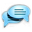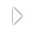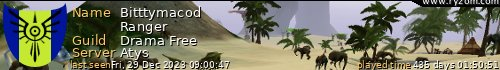How to locate A Ranger portal with just two flags - YOUR CREATIONS & GUIDES - Ryzom Community ForumGast1. . . and some math.

landmarks == flags

How to locate A Ranger portal with just two measurements

You thought that math in elementary school will never come handy?
Here it is!

You know there is a portal out there.
You know that the Ranger map with the amber can tell you within 500 meters where the portal is.
What it cannot tell you is what direction.
I am going to show you how to figure this out with just two flags.

You will need a piece of paper and pencil, and an arc-cosine table. Alternatively, you can use a spreadsheet like Microsoft Excel or LibreCalc;-)

If we know the length of the three sides of a triangle, any shaped triangle, we can tell each and every angle of it using arc-cosine.

The equation is as follows for each angle:
Let's make the length of each side named a, b, and c, while name the angles A, B, and C.

A=arccos(b^2+c^2-a^2 / 2bc)
B=arccos(a^2+c^2-b^2 / 2ac)
B=arccos(a^2+b^2-c^2 / 2ab)

Let's put this into use to find the portal.

Pull out your map, and let the amber search for the portal.
Your first result is say 78. That means the portal is 78 meters away in any direction.
Drop a flag and name the flag '78'.

Now start out and travel towards any direction.
Pull out your map, and let the amber search for the portal.
The second result is 113. The portal now is 113 meters away, you went the wrong direction.
Drop a flag and name it '113'. Do not move! If you moved off of the flag 113, move back to it until it is saying you are 0 meters away.

Target the flag 78. How far is it? In our example, it is 169 meters away.

Suddenly, now we have the three sides of the triangle.
a=78
b=113
c=169

Do not move! On your compass, right-click and select North. turn until you are facing North, as on the example. The white compass triangle points to the top.

Now, use your elementary school calculation and get the angle for angle B, or the point that is connecting side b to side c.

Put the numbers in your spreadsheet in cells A1, A2, and A3, respectively.

In Excel/LibreCalc/OpenCalc the equation is:
In C1 put the following equation. This calculates the angle for angle B, which is at the point where sides a and side c meet.

=DEGREES(ACOS((A2^2+A3^2-A1^2)/(2*A2*A3)))

My result for this angle is 33.92510046.... Which means nothing unless you have a protractor.

Take a look at 34 degrees. It appears twice on the protractor, on the left of 90 and right of the 90 degrees.
Since the other point is to the left of us, look at the left side.
From look at North (90 degrees), the portal is 34 degrees, and 113 meters away.
If we were at the left flag (flag 78), the calculation would be :

=DEGREES(ACOS((sidea^2+sideb^2-sidec^2)/(2*sidea*sideb)))

My result for that is 123.4160631. Since the other flag is to the right, the portal is 123 degrees, and 78 meters away.

To make it into step actions:

0. get closer than 500 meters to portal
1. measure first distance sidea to portal
2. create flag at current location. Name it the distance to the portal
3. run somewhere else
4. measure second distance sideb to portal
5. measure distance to first, sidea flag, this is sidec
6. look North
6. calculate angle from current spot using sidea, sideb and sidec measurements
=DEGREES(ACOS((sidea^2+sideb^2-sidec^2)/(2*sidea*sideb)))
7. turn to calculated angle and run sideb meters
9. portal should be under you

In our example, purple flags are the measurement spots, and white flag is portal.Hope this helps.

4 mal geändert | Zuletzt geändert von Nudge (vor 1 Jahre)There is one small problem with your analysis.

There are actually two points that satisfy all the conditions you specify. They are on either side of the line between A and B.
(Specifically the defined triangle can be flipped along the A-B line.)

It's usually enough to look at the two locations and realize that solution #2 is in an aggro pile and look at the other one. :D

If you find a third point you can get a unique solution. I tend to just target point B, estimate where I'm going to end up (based on my eyeballs on the map, then run until the distance to point B is the right number, then target point A, and iteratively adjust my distances until I'm there, but that's just because I'm too lazy to do the maths.

---Remembering Tyneetryk
Phaedreas Tears - 15 years old and first(*) of true neutral guilds in Atys.
(*) This statement is contested, but we are certainly the longest lasting.
<clowns | me & you | jokers>
There are actually more "problems" with the solution, like if the portal point is on the southern side of the circle, or if the angles are extremely acute, or the flags are in-line, and so on.

I did not want to make people bleed from their eyes.

As we all know Portals, TPs and such tend to be near natural and homin made boundary structure (cliffs, walls, rock faces, etc.) That should help with locating the portals.

Initially, I wrote up a quadrilateral solution, but then I realized, people will just hate me. You should have seen my complex quadrilaterals method. 8-)
Bitttymacod
There is one small problem with your analysis.

There are actually two points that satisfy all the conditions you specify. They are on either side of the line between A and B.
(Specifically the defined triangle can be flipped along the A-B line.)

It's usually enough to look at the two locations and realize that solution #2 is in an aggro pile and look at the other one. :D

If you find a third point you can get a unique solution. I tend to just target point B, estimate where I'm going to end up (based on my eyeballs on the map, then run until the distance to point B is the right number, then target point A, and iteratively adjust my distances until I'm there, but that's just because I'm too lazy to do the maths.Bitttymacod
There is one small problem with your analysis.

There are actually two points that satisfy all the conditions you specify. They are on either side of the line between A and B.
(Specifically the defined triangle can be flipped along the A-B line.)

It's usually enough to look at the two locations and realize that solution #2 is in an aggro pile and look at the other one. :D

If you find a third point you can get a unique solution. I tend to just target point B, estimate where I'm going to end up (based on my eyeballs on the map, then run until the distance to point B is the right number, then target point A, and iteratively adjust my distances until I'm there, but that's just because I'm too lazy to do the maths.

That's pretty much what I do. I use two flags, take a couple unflagged measurements to check which side of the line is the correct one, and check my distances from those flags once I figure out which side of the line I need to be on.

BTW, Ranger portals are usable even when a Kitin is trying to eat you; taking a few hundred HP worth of damage will not abort your travel the way it would with a normal teleport pact.

---

Do not assume that you speak for all just because you are the loudest voice; there are many who disagree that simply have no desire to waste words on you.
Last visit Mi 17 Jul 2019 14:40:41 UTC UTC
P_: